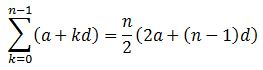# The Sum of the First n Terms of an Arithmetic Sequence

An error occurred trying to load this video.

Try refreshing the page, or contact customer support.

Coming up next: Understanding Arithmetic Series in Algebra

### You're on a roll. Keep up the good work!

Replay
Your next lesson will play in 10 seconds
• 0:02 An Arithmetic Sequence
• 0:59 The Sum Formula
• 2:00 Using the Formula
• 3:49 Another Example
• 5:16 Lesson Summary

Want to watch this again later?

Timeline
Autoplay
Autoplay
Speed

#### Recommended Lessons and Courses for You

Lesson Transcript
Instructor: Yuanxin (Amy) Yang Alcocer

Amy has a master's degree in secondary education and has taught math at a public charter high school.

In this video lesson, you will learn an easy to use formula for summing an arithmetic sequence. Watch as we use the formula in action and then get a chance to use it yourself as you follow along.

## An Arithmetic Sequence

Our video lesson begins with a quick overview of what an arithmetic sequence is. It is a string of numbers where the difference between each pair of successive numbers is the same. So, for example, our number line is an arithmetic sequence where the difference is 1 between each pair of the numbers. This difference between the pairs of numbers is referred to as the common difference. You can also think of an arithmetic sequence as telling you how much you have of something as it grows. Say you have a savings account at the bank. If you start with 10 dollars and you continue to put 10 dollars into the account every day, your account balance can be written as an arithmetic sequence where the difference between each pair of numbers is 10. We write our arithmetic sequence between curly brackets and with commas between the numbers. Writing our sequence for the bank, we have {10, 20, 30, 40, . . .}. We use the three dots at the end to show that the sequence goes on indefinitely.

## The Sum Formula

Sometimes, we want to add up the numbers in our sequence. For example, if our arithmetic sequence shows how many strawberries we are able to harvest each day, then adding up our sequence will tell us how many total strawberries we will have picked. To do this, we have a formula that will help us. The formula says that the sum of the first n terms of our arithmetic sequence is equal to n divided by 2 times the sum of twice the beginning term, a, and the product of d, the common difference, and n minus 1. The n stands for the number of terms we are adding together.The part of this formula that we will use is the part after the equals sign. The part before the equals sign just tells you that you are going to add each term. What this first part does is gives you a way to calculate each term in your sequence and then to sum it up. But since we are looking for what this sum equals, we only need to concern ourselves with the part after the equal sign.

## Using the Formula

To use this formula, we label our n, a, and d in our problem and then we plug the appropriate numbers in and evaluate. Let's see how this is done. Say we are the strawberry farmers, and we picked 20 strawberries the first day. This is strawberry season, so every day more and more strawberries are ripening. Each day, we are able to pick 5 more strawberries than the previous day. We can write this as an arithmetic sequence where the common difference is 5. We write {20, 25, 30, . . .}.

To find out how many strawberries we are able to pick after 30 days, we need to use our sum formula. We label our n as 30, our a as 20 (since that is our beginning number), and d as 5 (since that is our common difference). Now that everything is labeled, we can plug in these numbers and evaluate to get our answer. We plug our numbers into the part of the formula that is behind the equals sign. We get 30 divided by 2 times the sum of 2 times 20 and 30 minus 1 times 5.

To unlock this lesson you must be a Study.com Member.

### Register to view this lesson

Are you a student or a teacher?

### Unlock Your Education

#### See for yourself why 30 million people use Study.com

##### Become a Study.com member and start learning now.
Back
What teachers are saying about Study.com

### Earning College Credit

Did you know… We have over 160 college courses that prepare you to earn credit by exam that is accepted by over 1,500 colleges and universities. You can test out of the first two years of college and save thousands off your degree. Anyone can earn credit-by-exam regardless of age or education level.